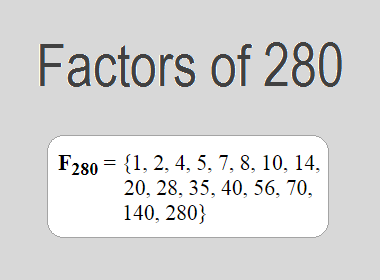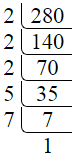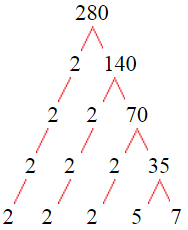# Factors of 280The factors of 280 are 1, 2, 4, 5, 7, 8, 10, 14, 20, 28, 35, 40, 56, 70, 140, and 280 i.e. F280 = {1, 2, 4, 5, 7, 8, 10, 14, 20, 28, 35, 40, 56, 70, 140, 280}. The factors of 280 are all the numbers that can divide 280 without leaving a remainder.

We can check if these numbers are factors of 280 by dividing 280 by each of them. If the result is a whole number, then the number is a factor of 280. Let's do this for each of the numbers listed above:

·        1 is a factor of 280 because 280 divided by 1 is 280.

·        2 is a factor of 280 because 280 divided by 2 is 140.

·        4 is a factor of 280 because 280 divided by 4 is 70.

·        5 is a factor of 280 because 280 divided by 5 is 56.

·        7 is a factor of 280 because 280 divided by 7 is 40.

·        8 is a factor of 280 because 280 divided by 8 is 35.

·        10 is a factor of 280 because 280 divided by 10 is 28.

·        14 is a factor of 280 because 280 divided by 14 is 20.

·        20 is a factor of 280 because 280 divided by 20 is 14.

·        28 is a factor of 280 because 280 divided by 28 is 10.

·        35 is a factor of 280 because 280 divided by 35 is 8.

·        40 is a factor of 280 because 280 divided by 40 is 7.

·        56 is a factor of 280 because 280 divided by 56 is 5.

·        70 is a factor of 280 because 280 divided by 70 is 4.

·        140 is a factor of 280 because 280 divided by 140 is 2.

·        280 is a factor of 280 because 280 divided by 280 is 1.

## How to Find Factors of 280?

1 and the number itself are the factors of every number. So, 1 and 280 are two factors of 280. To find the other factors of 280, we can start by dividing 280 by the numbers between 1 and 280. If we divide 280 by 2, we get a remainder of 0. Therefore, 2 is a factor of 280. If we divide 280 by 3, we get a remainder of 1. Therefore, 3 is not a factor of 280.

Next, we can check if 4 is a factor of 280. If we divide 280 by 4, we get a remainder of 0. Therefore, 4 is a factor of 280. We can continue this process for all the possible factors of 280.

Through this process, we can find that the factors of 280 are 1, 2, 4, 5, 7, 8, 10, 14, 20, 28, 35, 40, 56, 70, 140, and 280. These are the only numbers that can divide 280 without leaving a remainder.

********************

********************

## Properties of the Factors of 280

The factors of 280 have some interesting properties. One of the properties is that the sum of the factors of 280 is equal to 720. We can see this by adding all the factors of 280 together:

1 + 2 + 4 + 5 + 7 + 8 + 10 + 14 + 20 + 28 + 35 + 40 + 56 + 70 + 140 + 280 = 720

Another property of the factors of 280 is that the prime factors of 280 are 2, 5, and 7 only.

## Applications of the Factors of 280

The factors of 280 have several applications in mathematics. One of the applications is in finding the highest common factor (HCF) of two or more numbers. The HCF is the largest factor that two or more numbers have in common. For example, to find the HCF of 280 and 84, we need to find the factors of both numbers and identify the largest factor they have in common. The factors of 280 are 1, 2, 4, 5, 7, 8, 10, 14, 20, 28, 35, 40, 56, 70, 140, and 280. The factors of 84 are 1, 2, 3, 4, 6, 7, 12, 14, 21, 28, 42, and 84. The largest factor that they have in common is 28. Therefore, the HCF of 280 and 84 is 28.

Another application of the factors of 280 is in prime factorization. Prime factorization is the process of expressing a number as the product of its prime factors. The prime factors of 280 are 2, 5, and 7, since these are the only prime numbers that can divide 280 without leaving a remainder. Therefore, we can express 280 as:

280 = 2 × 2 × 2 × 5 × 7

We can do prime factorization by division and factor tree method also. Here is the prime factorization of 280 by division method,280 = 2 × 2 × 2 × 5 × 7

Here is the prime factorization of 280 by the factor tree method,280 = 2 × 2 × 2 × 5 × 7

## Conclusion

The factors of 280 are the numbers that can divide 280 without leaving a remainder. The factors of 280 are 1, 2, 4, 5, 7, 8, 10, 14, 20, 28, 35, 40, 56, 70, 140, and 280. The factors of 280 have some interesting properties, such as having a sum of 720. The factors of 280 have several applications in mathematics, such as finding the highest common factor and prime factorization.